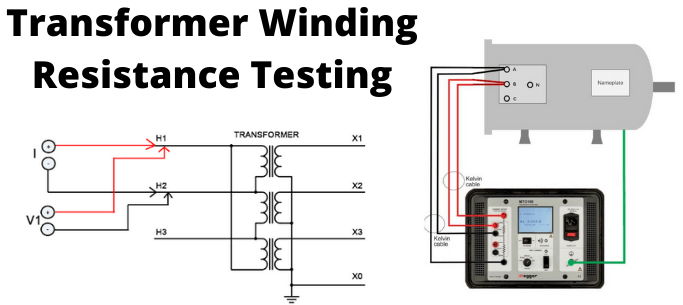# WINDING RESISTANCE TEST OF TRANSFORMER

Each transformer would be tested to determine its specifications by some tests as type test, routine test, special test.

In the factory, the main purposes of this test are as follows:

1. Calculating the I2R losses in the transformer.
2. Calculating winding temperature at the end of temperature rise test of transformer.
3. As a standard for assessing possible damages in the field.

It is carried out at the site in order to inspect abnormalities due to loosen connections, high contact resistance in tap changers, broken strands of conductors, high voltage leads, and bushings.

## Bridge Method of Measurement of Winding Resistance

The bridge method is based on comparing a known resistance with an unknown resistance. When currents flowing through the arms of the bridge circuit become balanced, the reading of the galvanometer shows zero deflection that means at a balanced condition no current will flow through the galvanometer.

A very small value of resistance (in milli-ohms range) can be accurately measured by the Kelvin bridge method whereas for higher value Wheatstone bridge method of resistance measurement is applied. In the bridge method of measurement of winding resistance, the errors are minimized.The resistance measured by Kelvin bridge,All other steps to be carried out during measuring winding resistance of transformer in these methods are similar to that of the current-voltage method of measuring transformer winding resistance, except the measuring technique of resistance.

The resistance measurement is done by Wheatstone bridge,

## The procedure of Measuring Transformer Winding Resistance

For star-connected winding, the resistance measurement shall be done between the neutral terminal and the line.

For star-connected autotransformers, the resistance measurement of the HV side is carried out between the IV terminal and the HV terminal, then between the neutral and IV terminal.
For delta-connected windings, winding resistance shall be measured between pairs of line terminals. As in delta connection, the resistance measurement of individual winding can not be done separately, the resistance per winding shall be calculated based on the following formula:

Resistance per winding = 1.5 × Measured value

The resistance measurement is carried out at an ambient temperature and then converted to resistance at 75oC for all practical purposes of comparison with specified design values, previous results, and diagnostics.

Winding Resistance at a standard temperature of 75oCIn which,

Rt = Winding resistance at temperature t
t = Winding temperature

In general, transformer windings are covered with paper insulation and immersed in insulation liquid, therefore it is impossible to measure the actual winding temperature in a de-energizing transformer at the time of transformer winding resistance measurement. An approximation is developed to calculate the temperature of winding at that condition, as follows:

The temperature of winding = Average temperature of insulating oil

The average temperature of insulating oil should be taken 3 to 8 hours after de-energizing the transformer and when the difference between the bottom and top oil temperatures becomes less than 5oC.

The resistance measurement can be done by a simple voltmeter ammeter method, Kelvin Bridge meter, or automatic winding resistance measurement kit (ohm meter, preferably 25 Amps kit).

Caution for voltmeter ammeter method: Current shall be less than 15% of the rated current of the winding. If it is larger, the heat of winding cause inaccuracy and thereby changing its temperature and resistance.

Note: The winding resistance of a transformer shall be measured at each tap.

## Current-Voltage Method of Measurement of Winding Resistance

The resistances measurement of the transformer winding can be done by the current-voltage method. In this method, the test current is applied to the winding and the corresponding voltage drop across the winding is measured.

According to simple Ohm’s law

i.e., Rx = V ⁄ I, determine the value of resistance can easily be determined.

## The procedure of Current-Voltage Method of Measurement of Winding Resistance

1. Before measurement, the winding temperature has to be equal to its oil temperature. And, the transformer should be in OFF condition without excitation at least for 3 to 4 hours.
2. Measurement is carried out with D.C.
3. The polarity of the core magnetization shall be constant to minimize observation errors during all resistance readings.
4. In order to protect Voltmeter leads from high voltages which may occur during switching on and off the current circuit, it shall be independent of the current leads
5. The readings shall be taken after the voltage and current have become steady-state values. In some cases, it spends several minutes depending upon the winding impedance.
6. The test current shall be less than 15% of the rated current of the winding. If it is larger, the heat of winding causes inaccuracy and thereby changing its resistance.
7. For expressing resistance, the resistance value must be mentioned along with the corresponding temperature of the winding at the time of measurement. As we said earlier that after remaining in switch-off condition for 3 to 4 hours, the oil temperature would be equal to the winding temperature. The oil temperature during testing is taken as the average of bottom oil and top oil temperatures of the transformer.1. For star-connected three-phase winding, the measured resistance between two-line terminals of the transformer would be 2 times the resistance per phase.
2. For delta-connected three-phase winding, measured resistance between two-line terminals of the transformer would be smaller than the resistance per phase.
3. This current-voltage method of measurement of winding resistance of transformer should be repeated for each pair of line terminals of winding at every tap position..(+84) 0913.006.538
Skype, Telegram, Wechat ...